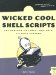28 Converting Temperatures

#28 Converting Temperatures

This script works with a variety of mathematical formulas, and an unusual input format, to translate between Fahrenheit, Celsius, and Kelvin. It's the first use of sophisticated mathematics within a script in this book, and you'll see where the experimentation in Script #9 that produced scriptbc proves a tremendous boon, as the same concept of piping an equation to bc shows up again here.

The Code

#!/bin/sh # convertatemp - Temperature conversion script that lets the user enter #   a temperature in Fahrenheit, Celsius, or Kelvin and receive the #   equivalent temperature in the other two units as the output. if [ \$# -eq 0 ] ; then   cat << EOF >&2 Usage:
#!/bin/sh # convertatemp - Temperature conversion script that lets the user enter # a temperature in Fahrenheit, Celsius, or Kelvin and receive the # equivalent temperature in the other two units as the output. if [ \$# -eq 0 ] ; then cat << EOF >&2 Usage: \$0 temperature[FCK] where the suffix: F indicates input is in Fahrenheit (default) C indicates input is in Celsius K indicates input is in Kelvin EOF exit 1 fi unit="\$(echo \$1sed -e 's/[-[[:digit:]]*//g'  tr '[:lower:]' '[:upper:]' )" temp="\$(echo \$1sed -e 's/[^-[[:digit:]]*//g')" case \${unit:=F} in F ) # Fahrenheit to Celsius formula: Tc = (F - 32) / 1.8 farn="\$temp" cels="\$(echo "scale=2;(\$farn - 32) / 1.8"  bc)" kelv="\$(echo "scale=2;\$cels + 273.15"  bc)" ;; C ) # Celsius to Fahrenheit formula: Tf = (9/5)*Tc+32 cels=\$temp kelv="\$(echo "scale=2;\$cels + 273.15"  bc)" farn="\$(echo "scale=2;((9/5) * \$cels) + 32"  bc)" ;; K ) # Celsius = Kelvin - 273.15, then use Cels -> Fahr formula kelv=\$temp cels="\$(echo "scale=2; \$kelv - 273.15"  bc)" farn="\$(echo "scale=2; ((9/5) * \$cels) + 32"  bc)" esac echo "Fahrenheit = \$farn" echo "Celsius = \$cels" echo "Kelvin = \$kelv" exit 0
temperature[FCK] where the suffix: F indicates input is in Fahrenheit (default) C indicates input is in Celsius K indicates input is in Kelvin EOF exit 1 fi unit="\$(echo sed -e 's/[-[[:digit:]]*//g' tr '[:lower:]' '[:upper:]' )" temp="\$(echo sed -e 's/[^-[[:digit:]]*//g')" case \${unit:=F} in F ) # Fahrenheit to Celsius formula: Tc = (F - 32) / 1.8 farn="\$temp" cels="\$(echo "scale=2;(\$farn - 32) / 1.8" bc)" kelv="\$(echo "scale=2;\$cels + 273.15" bc)" ;; C ) # Celsius to Fahrenheit formula: Tf = (9/5)*Tc+32 cels=\$temp kelv="\$(echo "scale=2;\$cels + 273.15" bc)" farn="\$(echo "scale=2;((9/5) * \$cels) + 32" bc)" ;; K ) # Celsius = Kelvin - 273.15, then use Cels -> Fahr formula kelv=\$temp cels="\$(echo "scale=2; \$kelv - 273.15" bc)" farn="\$(echo "scale=2; ((9/5) * \$cels) + 32" bc)" esac echo "Fahrenheit = \$farn" echo "Celsius = \$cels" echo "Kelvin = \$kelv" exit 0

Running the Script

I really like this script because I like the intuitive nature of the input format, even if it is pretty unusual for a Unix command. Input is entered as a numeric value, with an optional suffix that indicates the units of the temperature entered. To see the Celsius and Kelvin equivalents of the temperature 100 degrees Fahrenheit, enter 100F. To see what 100 degrees Kelvin is equivalent to in Fahrenheit and Celsius, use 100K. If no unit suffix is entered, this script works with Fahrenheit temperatures by default.

You'll see this same logical single-letter suffix approach again in Script #66, which converts currency values.

The Results

\$  convertatemp 212  Fahrenheit = 212 Celsius    = 100.00 Kelvin     = 373.15 \$  convertatemp 100C  Fahrenheit = 212.00 Celsius    = 100 Kelvin     = 373.15 \$  convertatemp 100K  Fahrenheit = -279.67 Celsius    = -173.15 Kelvin     = 100

Hacking the Script

A few input flags that would generate a succinct output format suitable for use in other scripts would be a useful addition to this script. Something like convertatemp -c 100f could output the Celsius equivalent of 100 degrees Fahrenheit.Wicked Cool Shell Scripts
ISBN: 1593270127
EAN: 2147483647
Year: 2004
Pages: 150
Authors: Dave Taylor

Similar book on Amazon